# Pandas GroupBy – Count occurrences in column

Using the size() or count() method with pandas.DataFrame.groupby() will generate the count of a number of occurrences of data present in a particular column of the dataframe. However, this operation can also be performed using pandas.Series.value_counts() and, pandas.Index.value_counts().

Approach

• Import module
• Create or import data frame
• Apply groupby
• Use any of the two methods
• Display result

### Method 1: Using pandas.groupyby().size()

The basic approach to use this method is to assign the column names as parameters in the groupby() method and then using the size() with it. Below are various examples that depict how to count occurrences in a column for different datasets.

Example 1:

In this example, we separately count occurrences of all the columns present in a dataset.

## Python3

 `# import module` `import` `pandas as pd`     `# assign data` `data ``=` `pd.DataFrame({``'Section'``: [``'A'``, ``'A'``, ``'A'``, ``'B'``, ``'B'``,` `                                 ``'B'``, ``'C'``, ``'C'``, ``'C'``],` `                     ``'Teacher'``: [``'Kakeshi'``, ``'Kakeshi'``, ``'Iruka'``,` `                                 ``'Kakeshi'``, ``'Kakeshi'``, ``'Kakeshi'``,` `                                 ``'Iruka'``, ``'Iruka'``, ``'Guy'``]})`   `# display dataframe` `print``(``'Data:'``)` `display(data)`     `print``(``'Occurrence counts of particular columns:'``)`   `# count occurrences a particular column` `occur ``=` `data.groupby([``'Section'``]).size()`   `# display occurrences of a particular column` `display(occur)`   `# count occurrences a particular column` `occur ``=` `data.groupby([``'Teacher'``]).size()`   `# display occurrences of a particular column` `display(occur)`

Output: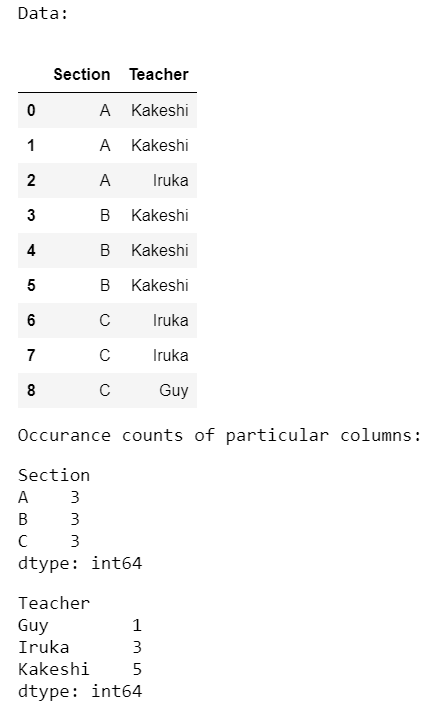Example 2:

In the below program, we count occurrences of all the columns combined from the same dataset as used in the previous program.

## Python3

 `# import module` `import` `pandas as pd`     `# assign data` `data ``=` `pd.DataFrame({``'Section'``: [``'A'``, ``'A'``, ``'A'``, ``'B'``, ``'B'``, ``'B'``,` `                                 ``'C'``, ``'C'``, ``'C'``],` `                     ``'Teacher'``: [``'Kakeshi'``, ``'Kakeshi'``, ``'Iruka'``,` `                                 ``'Kakeshi'``, ``'Kakeshi'``, ``'Kakeshi'``,` `                                 ``'Iruka'``, ``'Iruka'``, ``'Guy'``]})`   `# display dataframe` `print``(``'Data:'``)` `display(data)`     `print``(``'Occurrence counts of combined columns:'``)`   `# count occurrences of combined columns` `occur ``=` `data.groupby([``'Section'``, ``'Teacher'``]).size()`   `# display occurrences of combined columns` `display(occur)`

Output: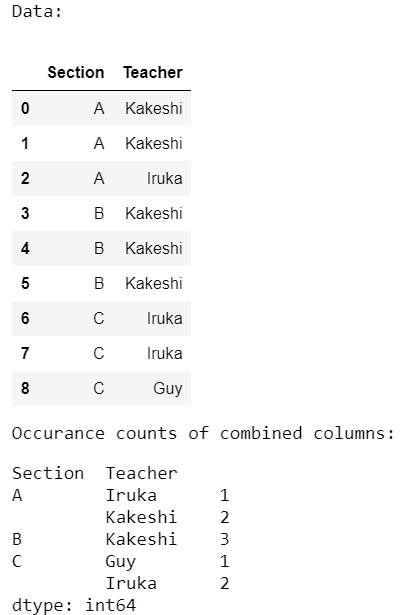Example 3:

Here, we separate count occurrences and combined count occurrences of the categorical columns present in a CSV file.

## Python3

 `# import module` `import` `pandas as pd`     `# assign data` `data ``=` `pd.read_csv(``'diamonds.csv'``)`   `# display dataframe` `print``(``'Data:'``)` `display(data.sample(``10``))`   `print``(``'Occurrence counts of particular column:'``)`   `# count occurrences a particular column` `occur ``=` `data.groupby([``'cut'``]).size()`   `# display occurrences of a particular column` `display(occur)`   `print``(``'Occurrence counts of combined columns:'``)`   `# count occurrences of combined columns` `occur ``=` `data.groupby([``'clarity'``, ``'color'``, ``'cut'``]).size()`   `# display occurrences of combined columns` `display(occur)`

Output: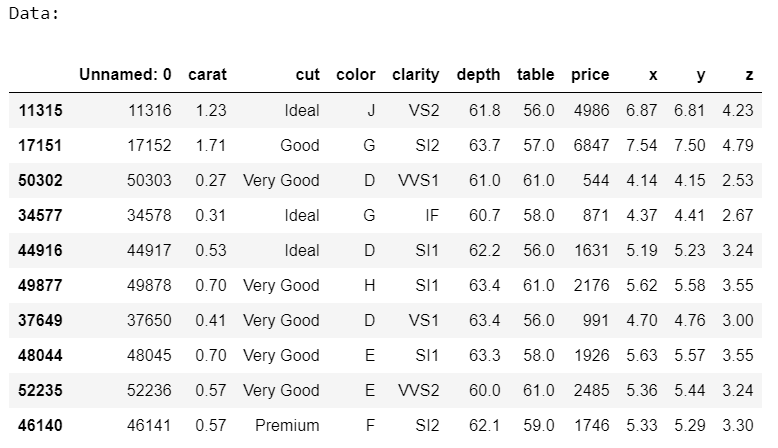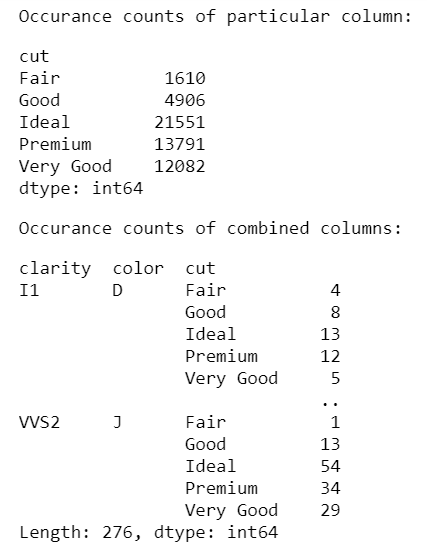### Method 2: Using pandas.groupyby().count()

The basic approach to use this method is to assign the column names as parameters in the groupby() method and then using the count() with it. Below are various examples that depict how to count occurrences in a column for different datasets.

Example 1:

In this example, we separately count occurrences of all the columns present in a dataset.

## Python3

 `# import module` `import` `pandas as pd`     `# assign data` `data ``=` `pd.DataFrame({``'Section'``: [``'A'``, ``'A'``, ``'A'``, ``'B'``, ``'B'``, ``'B'``,` `                                 ``'C'``, ``'C'``, ``'C'``],` `                     ``'Teacher'``: [``'Kakeshi'``, ``'Kakeshi'``, ``'Iruka'``,` `                                 ``'Kakeshi'``, ``'Kakeshi'``, ``'Kakeshi'``,` `                                 ``'Iruka'``, ``'Iruka'``, ``'Guy'``]})`   `# display dataframe` `print``(``'Data:'``)` `display(data)`   `print``(``'Occurrence counts of particular columns:'``)`   `# count occurrences a particular column` `occur ``=` `data.groupby([``'Section'``]).size()`   `# display occurrences of a particular column` `display(occur)`   `# count occurrences a particular column` `occur ``=` `data.groupby([``'Teacher'``]).size()`   `# display occurrences of a particular column` `display(occur)`

Output: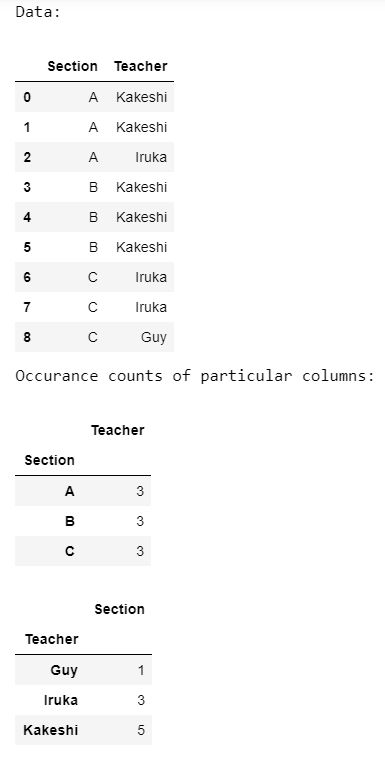Example 2:

In the below program, we count occurrences of all the columns combined from the same dataset as used in the previous program.

## Python3

 `# import module` `import` `pandas as pd`     `# assign data` `data ``=` `pd.DataFrame({``'Section'``: [``'A'``, ``'A'``, ``'A'``, ``'B'``, ``'B'``, ``'B'``,` `                                 ``'C'``, ``'C'``, ``'C'``],` `                     ``'Teacher'``: [``'Kakeshi'``, ``'Kakeshi'``, ``'Iruka'``,` `                                 ``'Kakeshi'``, ``'Kakeshi'``, ``'Kakeshi'``,` `                                 ``'Iruka'``, ``'Iruka'``, ``'Guy'``]})`   `# display dataframe` `print``(``'Data:'``)` `display(data)`   `print``(``'Occurrence counts of combined columns:'``)`   `# count occurrences of combined columns` `occur ``=` `data.groupby([``'Section'``, ``'Teacher'``]).size()`   `# display occurrences of combined columns` `display(occur)`

Output:Example 3:

Here, we separate count occurrences and combined count occurrences of the categorical columns present in a CSV file.

## Python3

 `# import module` `import` `pandas as pd`     `# assign data` `data ``=` `pd.read_csv(``'diamonds.csv'``)`   `# display dataframe` `print``(``'Data:'``)` `display(data.sample(``10``))`     `print``(``'Occurrence counts of particular column:'``)`   `# count occurrences a particular column` `occur ``=` `data.groupby([``'cut'``]).size()`   `# display occurrences of a particular column` `display(occur)`     `print``(``'Occurrence counts of combined columns:'``)`   `# count occurrences of combined columns` `occur ``=` `data.groupby([``'clarity'``, ``'color'``, ``'cut'``]).size()`   `# display occurrences of combined columns` `display(occur)`

Output: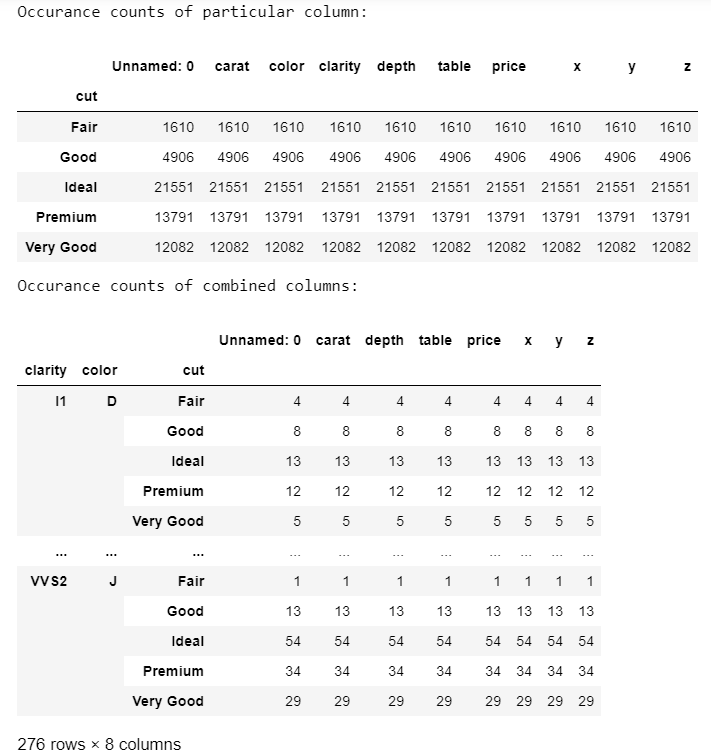Whether you're preparing for your first job interview or aiming to upskill in this ever-evolving tech landscape, GeeksforGeeks Courses are your key to success. We provide top-quality content at affordable prices, all geared towards accelerating your growth in a time-bound manner. Join the millions we've already empowered, and we're here to do the same for you. Don't miss out - check it out now!

Previous
Next